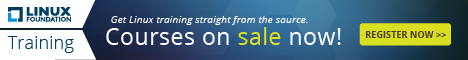<< View All Computer Tips

Added On: 18/12/15   |    Category: PHP5    |    Number of Views: 1836

## Convert a Unix timestamp to date and time using PHP    Tweet

To convert a unix timestamp to date and time using php we use the php date() and time() functions

The time() function returns the current time as a Unix timestamp (the number of seconds elapsed since January 1 1970 00:00:00 GMT).

In our code below we are displaying the date and time in the following format
(d=day of the month)(F=month)(Y=year, 4 digits) - (G=hour)(i=minutes)(s=seconds)(a=am or pm)

<?php
echo \$date = date("d F Y - G:i:s a",time());
?>

If you wanted to grab the time in seconds from your database you would use the following code
<?php
echo \$date = date("d F Y - G:i:s a",\$row['field_name']);
?>